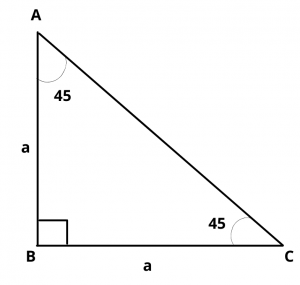# What is the Value of Tan 45 Degrees ?

## Solution :

The value of tan 45 degrees is 1.

Proof :

Let ABC be a triangle, right angled at B, in which $$\angle$$ A = $$\angle$$ C = 45 degrees$$\therefore$$  BC = AB

Let  AB = BC = a

Then by pythagoras theorem,

$$AC^2$$ = $$AB^2$$ + $$BC^2$$ = $$a^2$$ + $$a^2$$ = $$2a^2$$

$$\implies$$  AC = $$\sqrt{2}a$$

In $$\Delta$$ ABC,  $$\angle$$ C = 45 degrees

By using trigonometric formulas,

$$tan 45^{\circ}$$ = $$perpendicular\over base$$ = $$p\over b$$

$$tan 45^{\circ}$$  = side opposite to 45 degrees/side adjacent to 45 degrees = $$AB\over BC$$ = $$a\over a$$ = 1

Hence, the value of $$tan 45^{\circ}$$ = 1